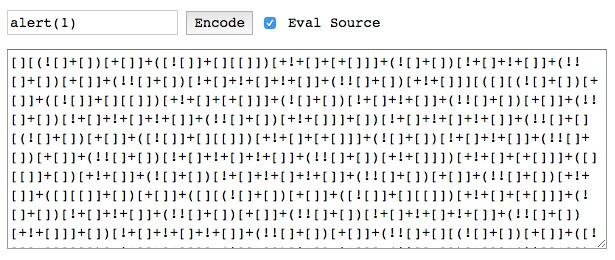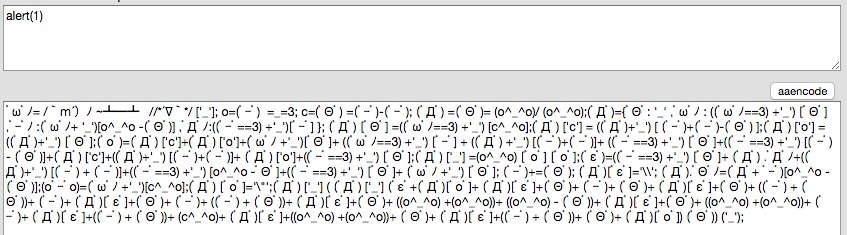# 讓 JavaSript 難以閱讀：jsfuck 與 aaencode

## 先從 Brainfuck 開始

1. >
2. <
3. +
4. -
5. .
6. ,
7. [
8. ]

1. ++ptr;
2. --ptr;
3. ++*ptr;
4. --*ptr;
5. putchar(*ptr);
6. *ptr =getchar();
7. while (*ptr) {
8. }

（資料來源：wikipedia: Brainfuck
Brainfuck 內建會給你一組陣列，並且讓 ptr 指向陣列開頭，剩下的事情就交給我們自己了，舉例來說，輸出 Hello World 的程式長這樣：

++++++++++[>+++++++>++++++++++>+++>+<<<<-]
>++.>+.+++++++..+++.>++.<<+++++++++++++++.
>.+++.------.--------.>+.>.


## JSfuck1. [
2. ]
3. (
4. )
5. !
6. +

## Function Constructor

new Function('alert(1)')();


new Function('a', 'b', 'return a+b;')(1, 2);
// 3


## 如何湊出程式碼？

+[]可以湊出 0，或者也可以換一個思路，![]會是 false，所以+![]也會是 0，有 0 之後，要變出 1 就不難了，因為 ![]false，所以 !![] 就是 true。那 +!![] 就是數字的 1。

## 把上面結合起來

[]['map']['constructor']


## 用顏文字寫程式aaencodeJSfuck 又有一點小差異了，因為aaencode可以用到的字元比較多，只是長得比較可愛而已，那既然JSfuck可以做到，aaencode沒什麼理由做不到。

（因為有些特殊字元的關係，可能會顯示不出來，但不影響整體的閱讀，看個感覺就好）

ﾟωﾟﾉ= /｀ｍ´）ﾉ ~┻━┻   //*´∇｀*/ ['_']; o=(ﾟｰﾟ)  =_=3;
c=(ﾟΘﾟ) =(ﾟｰﾟ)-(ﾟｰﾟ); (ﾟДﾟ) =(ﾟΘﾟ)= (o^_^o)/ (o^_^o);
(ﾟДﾟ)={ﾟΘﾟ: '_' ,ﾟωﾟﾉ : ((ﾟωﾟﾉ==3) +'_') [ﾟΘﾟ] ,ﾟｰﾟﾉ :(ﾟωﾟﾉ+ '_')[o^_^o -(ﾟΘﾟ)]
,ﾟДﾟﾉ:((ﾟｰﾟ==3) +'_') [ﾟｰﾟ] }; (ﾟДﾟ) [ﾟΘﾟ] =((ﾟωﾟﾉ==3) +'_') [c^_^o];(ﾟДﾟ) ['c'] =
((ﾟДﾟ) +'_') [ (ﾟｰﾟ)+(ﾟｰﾟ)-(ﾟΘﾟ) ];(ﾟДﾟ) ['o'] = ((ﾟДﾟ)+'_') [ﾟΘﾟ];(ﾟoﾟ)=(ﾟДﾟ)  ['c']+
(ﾟДﾟ) ['o']+(ﾟωﾟﾉ +'_')[ﾟΘﾟ]+ ((ﾟωﾟﾉ==3) +'_') [ﾟｰﾟ] + ((ﾟДﾟ)   +'_') [(ﾟｰﾟ)+(ﾟｰﾟ)]+ (
(ﾟｰﾟ==3) +'_') [ﾟΘﾟ]+((ﾟｰﾟ==3) +'_') [(ﾟｰﾟ) - (ﾟΘﾟ)]+(ﾟДﾟ) ['c']+((ﾟДﾟ)+'_') [(ﾟｰﾟ)+(
ﾟｰﾟ)]+ (ﾟДﾟ) ['o']+((ﾟｰﾟ==3) +'_')    [ﾟΘﾟ];(ﾟДﾟ) ['_'] =(o^_^o) [ﾟoﾟ] [ﾟoﾟ];(ﾟεﾟ)=((
ﾟｰﾟ==3) +'_') [ﾟΘﾟ]+ (ﾟДﾟ) .ﾟДﾟﾉ+((ﾟДﾟ)+'_') [(ﾟｰﾟ) + (ﾟｰﾟ)]+((ﾟｰﾟ==3) +'_') [o^_^o -
ﾟΘﾟ]+    ((ﾟｰﾟ==3) +'_') [ﾟΘﾟ]+ (ﾟωﾟﾉ +'_') [ﾟΘﾟ]; (ﾟｰﾟ)+=(ﾟΘﾟ); (ﾟДﾟ)[ﾟεﾟ]='\\'; (
ﾟДﾟ).ﾟΘﾟﾉ=(ﾟДﾟ+ ﾟｰﾟ)[o^_^o -(ﾟΘﾟ)];(oﾟｰﾟo)=(ﾟωﾟﾉ +'_')[c^_^o];(ﾟДﾟ)     [ﾟoﾟ]='\"';(
ﾟДﾟ) ['_'] ( (ﾟДﾟ) ['_'] (ﾟεﾟ+(ﾟДﾟ)[ﾟoﾟ]+ (ﾟДﾟ)[ﾟεﾟ]+(ﾟΘﾟ)+ (ﾟｰﾟ)+ (ﾟΘﾟ)+ (ﾟДﾟ)[ﾟεﾟ]+(
ﾟΘﾟ)+ ((ﾟｰﾟ) + (ﾟΘﾟ))+ (ﾟｰﾟ)+ (ﾟДﾟ)[ﾟεﾟ]+(ﾟ    Θﾟ)+ (ﾟｰﾟ)+ ((ﾟｰﾟ) + (ﾟΘﾟ))+ (ﾟДﾟ)[ﾟεﾟ]+
(ﾟΘﾟ)+ ((o^_^o) +(o^_^o))+     ((o^_^o) - (ﾟΘﾟ))+ (ﾟДﾟ)[ﾟεﾟ]+(ﾟΘﾟ)+ ((o^_^o) +(
o^_^o))+ (ﾟｰﾟ)+ (ﾟДﾟ)[ﾟεﾟ]+((ﾟｰﾟ) + (ﾟΘﾟ))+ (c^_^o)+ (ﾟДﾟ)[ﾟεﾟ]+((o^_^o) +(o^_^o))+ (
ﾟΘﾟ)+ (ﾟДﾟ)[ﾟεﾟ]+((ﾟｰﾟ) + (ﾟΘﾟ))+ (ﾟΘﾟ)+ (ﾟДﾟ)[ﾟoﾟ]) (ﾟΘﾟ)) ('_');


（底下的程式碼因為特殊字元的關係有多做一點處理，跟原本的有些許差異）

o=（˙_˙）  =_=3;


o = （˙_˙）   =  _  = 3;


_ = 3;
（˙_˙） = _;
o = （˙_˙）  ;


## 結論

@huli 野生工程師，相信分享與交流能讓世界變得更美好